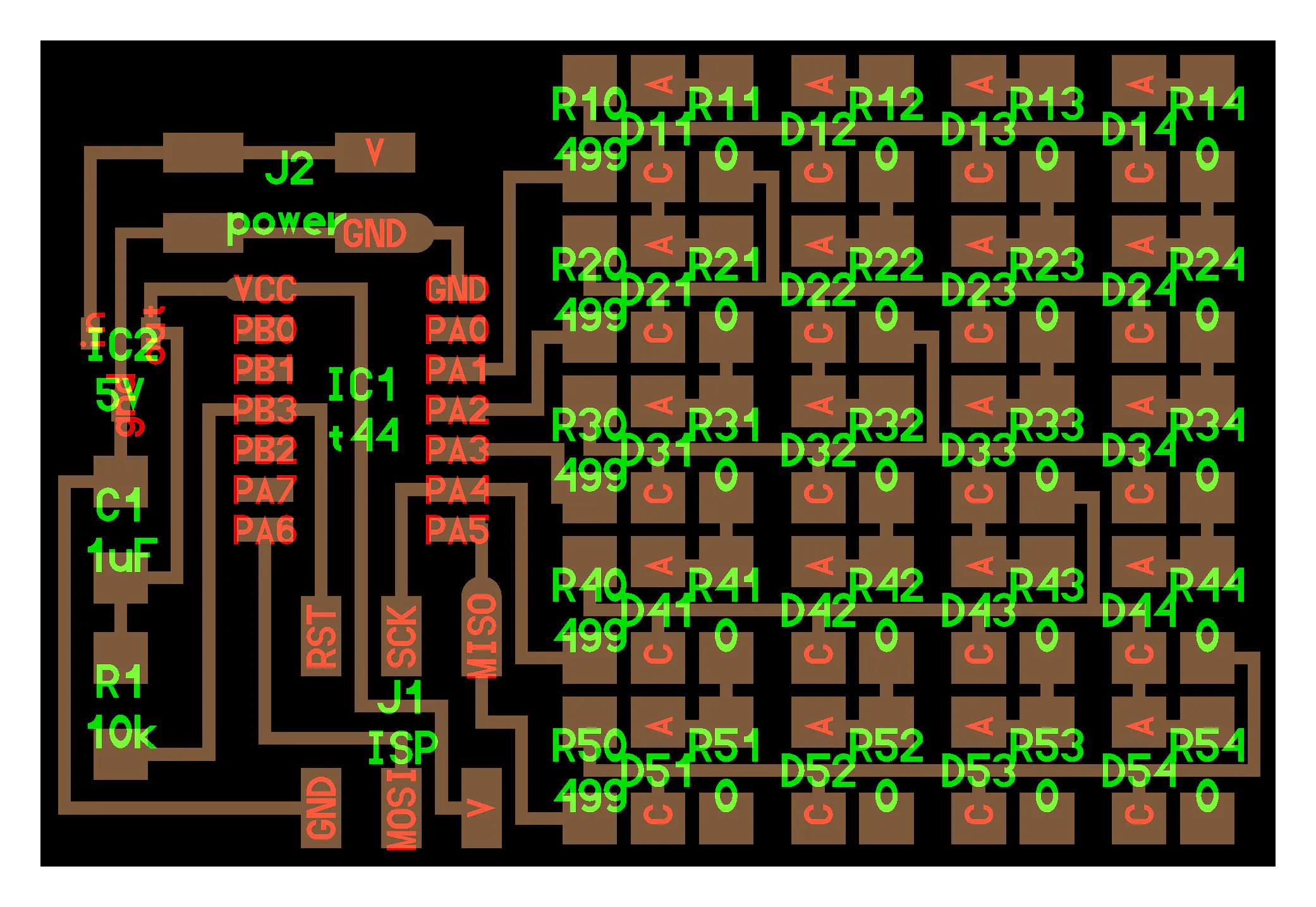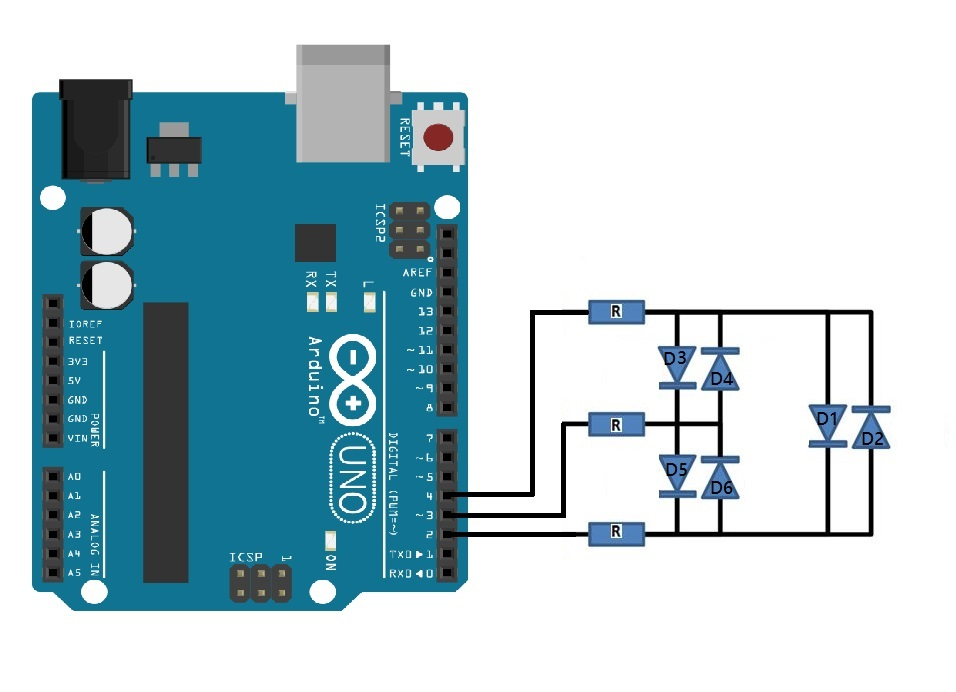Project tutorial# Charlieplexing with Arduino © GPL3+

Charlieplexing extends the limitation of I/O pins of a controller beyond its capability.

• 6,873 views
• 1 comment
• 11 respects

## Necessary tools and machines

 Arduino CC

Charlieplexing is that driving lots of LEDs with only a few pins. And in this laboratory experiment we drive 6 LEDs using 3 pins. This method of Charlieplexing uses the tri-state logic capabilities of micro-controllers in order to gain efficiency over traditional multiplexing. This is another form of multiplexing.

As per the above given to the charlieplexed display the following set of routines were written. The following program worked according to the requirement where the LED blinked in the pattern starting from LED1 to LED6.

The formula for Charlieplexing is:

Where n is the number of pins used.

A lookup table was drawn out to identify the pin pattern to light up each LED individually.

PDDR: Port Data Driven Register.

A: Pin 2

B: Pin 3

C: Pin 4

## Code

##### Arduino Code for CharlieplexingC/C++
```int A = 2;
int B = 3;
int C = 4;

void setup()
{
pinMode(A, OUTPUT);
pinMode(B, OUTPUT);
pinMode(C, OUTPUT);

}

/*
* Turn on the given LED
*
* @paramledNum LED to turn on (1..6)
*
*/
void setLED(int ledNum)
{
if(ledNum == 1)
{
pinMode(A, OUTPUT);
pinMode(B, INPUT);  // changed to input to trigger tri state.
pinMode(C, OUTPUT);

digitalWrite(A, HIGH);
digitalWrite(C, LOW);
}

if(ledNum == 2)
{
pinMode(A, OUTPUT);
pinMode(B, INPUT);
pinMode(C, OUTPUT);

digitalWrite(A, LOW);
digitalWrite(C, HIGH);
}

if(ledNum == 3)
{
pinMode(A, OUTPUT);
pinMode(B, OUTPUT);
pinMode(C, INPUT);

digitalWrite(A, HIGH);
digitalWrite(B, LOW);
}

if(ledNum == 4)
{
pinMode(A, OUTPUT);
pinMode(B, OUTPUT);
pinMode(C, INPUT);

digitalWrite(A, LOW);
digitalWrite(B,HIGH);
}

if(ledNum == 5)
{

pinMode(A, INPUT);
pinMode(B, OUTPUT);
pinMode(C, OUTPUT);

digitalWrite(B, HIGH);
digitalWrite(C, LOW);
}

if(ledNum == 6)
{

pinMode(A, INPUT);
pinMode(B, OUTPUT);
pinMode(C, OUTPUT);

digitalWrite(B, LOW);
digitalWrite(C, HIGH);
}
}

void loop()
{

for(;;)
{
setLED(1);
delay(500);
setLED(2);
delay(500);
setLED(3);
delay(500);
setLED(4);
delay(500);
setLED(5);
delay(500);
setLED(6);
delay(500);
}

}
```

## Schematics#### Arduino and AC Devices - Automatic Lights

Project tutorial by Ahmed Hamdy Mahmoud Hassanein

• 40,761 views
• 74 respects

#### Arduino Bluetooth Basic Tutorial

• 869,686 views
• 488 respects

#### Windows Remote Arduino

Project tutorial by Team Windows IoT

• 216,227 views
• 384 respects

#### Simple Programmable Robotic Arm

Project showcase by Ryan Chan

• 165,434 views
• 378 respects

#### 4x4x4 LED cube with Arduino Uno and 1sheeld

Project tutorial by Hassan Ibrahim

• 42,690 views